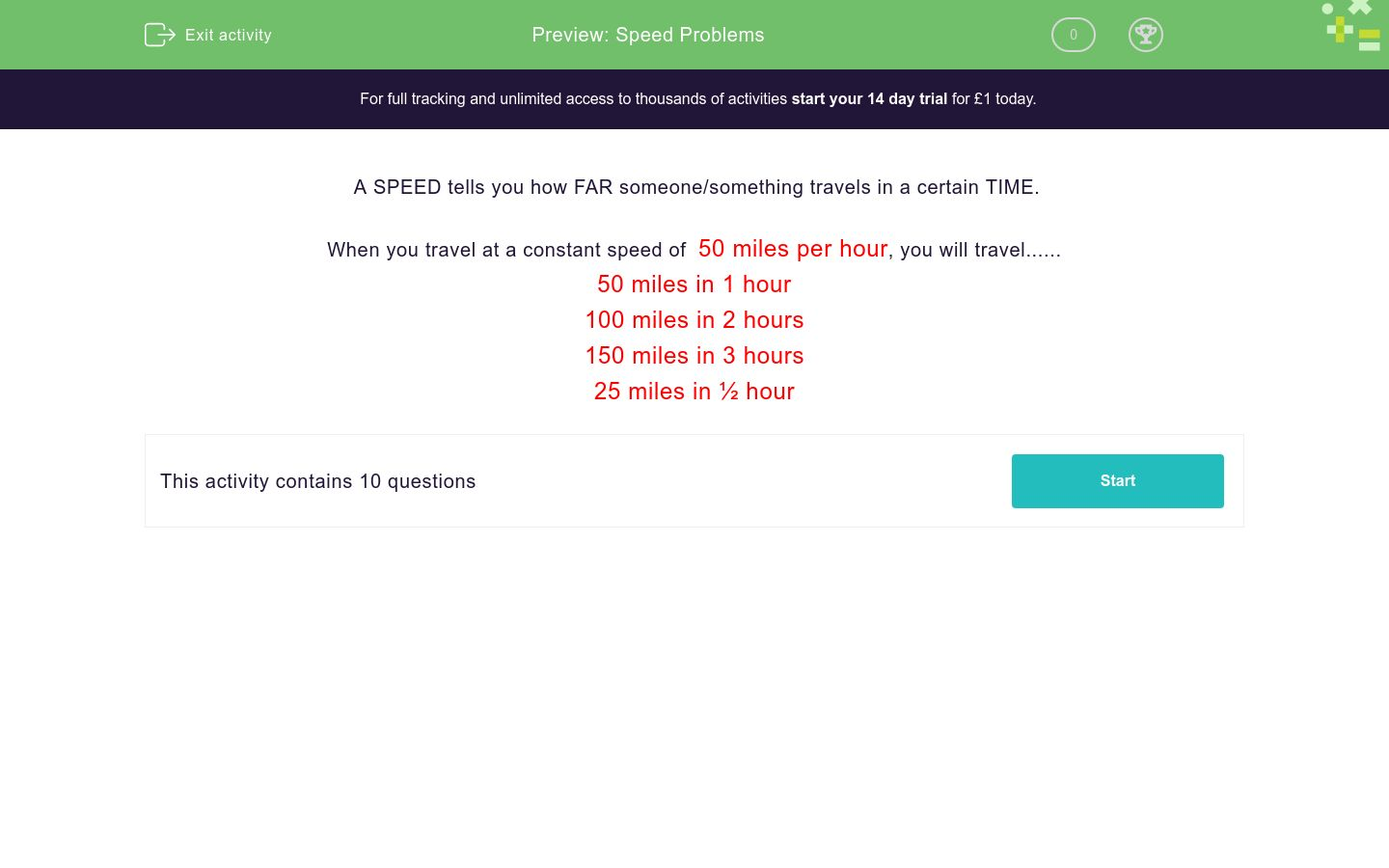# Speed Problems

In this worksheet, students answer questions based on simple speeds, distances and times.Key stage:  KS 2

Curriculum topic:   Number: Multiplication and Division

Curriculum subtopic:   Solve Multiplication/Division Problems

Difficulty level:### QUESTION 1 of 10

A SPEED tells you how FAR someone/something travels in a certain TIME.

When you travel at a constant speed of  50 miles per hour, you will travel......

50 miles in 1 hour

100 miles in 2 hours

150 miles in 3 hours

25 miles in ½ hour

Jack drives to London at a constant speed of 40 miles per hour.

How far will he travel in miles in 1 hour?

(just write the number)

Jack drives to London at a constant speed of 40 miles per hour.

How far will he travel in miles in 2 hours?

(just write the number)

Jack drives to London at a constant speed of 40 miles per hour.

How far will he travel in miles in ½ hour?

(just write the number)

Jill walks at a constant speed of 6 kilometres per hour.

How far will she walk in km in 3 hours?

(just write the number)

Jill walks at a constant speed of 6 kilometres per hour.

How far will she walk in km in ½ hour?

(just write the number)

Jill walks at a constant speed of 6 kilometres per hour.

How far will she walk in km in 1½ hours?

(just write the number)

Ahmed runs at a constant speed of 16 kilometres per hour.

How far will he run in km in 2 hours?

(just write the number)

Ahmed runs at a constant speed of 16 kilometres per hour.

How far will he run in km in ¼ hour?

(just write the number)

Ahmed runs at a constant speed of 16 kilometres per hour.

How many minutes will it take him to run 8 km?

(just write the number)

Ahmed runs at a constant speed of 16 kilometres per hour.

How many minutes will it take him to run 32 km?

(just write the number)

• Question 1

Jack drives to London at a constant speed of 40 miles per hour.

How far will he travel in miles in 1 hour?

(just write the number)

40
EDDIE SAYS
Jack travels 40 miles in 1 hour. 40 x 1 = 40
• Question 2

Jack drives to London at a constant speed of 40 miles per hour.

How far will he travel in miles in 2 hours?

(just write the number)

80
EDDIE SAYS
Jack travels 40 miles in 1 hour, so he travels 80 miles in 2 hours. 40 x 2 = 80
• Question 3

Jack drives to London at a constant speed of 40 miles per hour.

How far will he travel in miles in ½ hour?

(just write the number)

20
EDDIE SAYS
Jack travels 40 miles in 1 hour, so in 1/2 an hour he travels 20 miles. 1/2 of 40 = 40 divided by 2 = 20
• Question 4

Jill walks at a constant speed of 6 kilometres per hour.

How far will she walk in km in 3 hours?

(just write the number)

18
EDDIE SAYS
Jill walks 6km in 1 hour, so she walks 18km in 3 hours. 6 x 3 = 18
• Question 5

Jill walks at a constant speed of 6 kilometres per hour.

How far will she walk in km in ½ hour?

(just write the number)

3
EDDIE SAYS
Jill walks 6km in 1 hour, so in 1/2 an hour she walks 3km. 1/2 of 6 = 6 divided by 2 = 3
• Question 6

Jill walks at a constant speed of 6 kilometres per hour.

How far will she walk in km in 1½ hours?

(just write the number)

9
EDDIE SAYS
Jill walks 6km in 1 hour, so she walks 9km in 1 1/2 hours. 6km x 1 = 6km 1/2 of 6km = 3km 6 + 3 = 9
• Question 7

Ahmed runs at a constant speed of 16 kilometres per hour.

How far will he run in km in 2 hours?

(just write the number)

32
EDDIE SAYS
Ahmed runs 16km in 1 hour, so he runs 32km in 2 hours. 16 x 2 = 32
• Question 8

Ahmed runs at a constant speed of 16 kilometres per hour.

How far will he run in km in ¼ hour?

(just write the number)

4
EDDIE SAYS
Ahmed runs 16km in 1 hour, so he runs 4km in 1/4 of an hour. 1/4 of 16 = 16 divided by 4 = 4
• Question 9

Ahmed runs at a constant speed of 16 kilometres per hour.

How many minutes will it take him to run 8 km?

(just write the number)

30
EDDIE SAYS
Ahmed runs 16km in 1 hour, or 60 minutes, so it will take him 30 minutes to run 8km. 1/2 of 60 = 60 divided by 2 = 30
• Question 10

Ahmed runs at a constant speed of 16 kilometres per hour.

How many minutes will it take him to run 32 km?

(just write the number)

120
EDDIE SAYS
Ahmed runs 16km in 1 hour, or 60 minutes, so it will take him 2 hours, or 120 minutes, to run 32km. 60 minutes x 2 = 120 minutes
---- OR ----

Sign up for a £1 trial so you can track and measure your child's progress on this activity.

### What is EdPlace?

We're your National Curriculum aligned online education content provider helping each child succeed in English, maths and science from year 1 to GCSE. With an EdPlace account you’ll be able to track and measure progress, helping each child achieve their best. We build confidence and attainment by personalising each child’s learning at a level that suits them.

Get started